1年前   阅读数 222 0

# 200道大数据面试常考Leetcode算法题06-Z字形变换

Leetcode原题为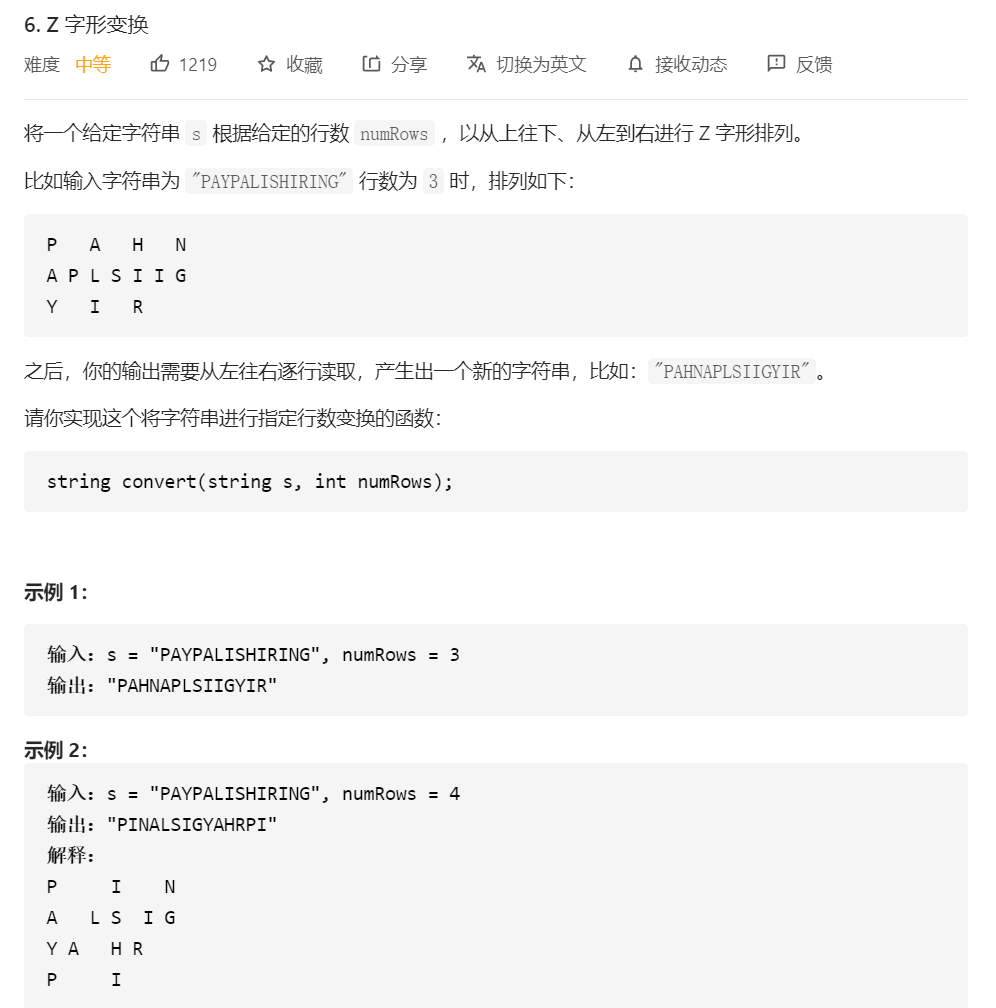``````class Solution:
def convert(self, s: str, numRows: int) -> str:
n = numRows * 2 - 2  # 判断z字形循环的周期规律为：行数*2减去头尾两个
if numRows < 2:  # 判断给出的z字形行数小于2时，z字形无法成型
return s   # 返回原数组，做安全容错处理
result = [""]*numRows  # 用一个["","",""]装纳每行的数据，这里是三行
for i, char in enumerate(s):  # 循环每个索引跟值
index = i % n     # 当前字符的索引整除周期的值
x = min(index, n - index)  # 找出最小的值，周期有峰值
result[x] += char   # 把当前的最小的索引值为行号，然后把当前值添加到当前索引行
return "".join(result)  # 最后3行字符拼接在一起``````

# 200道大数据面试常考Leetcode算法题07-整数反转

Leetcode原题：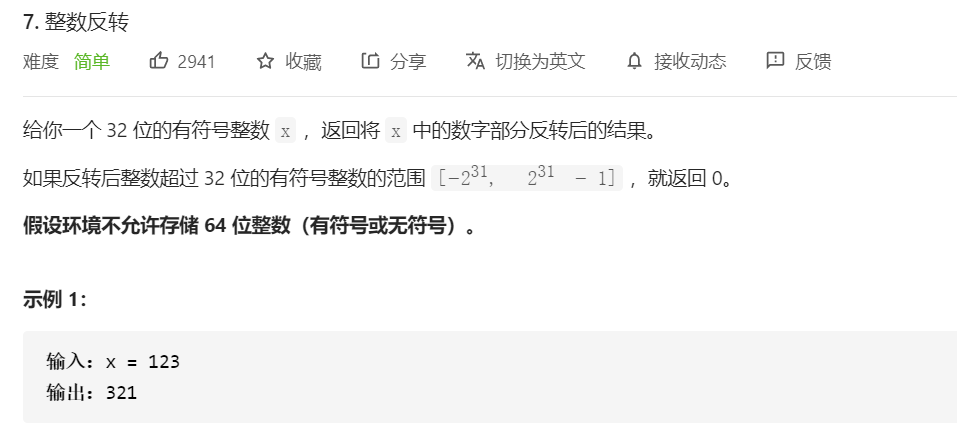``````class Solution:
def reverse(self, x: int) -> int:
# 判断对正数反转时
if x>=0:
# 整型变成字符串用切片，-1表示反转
ans=int(str(x)[::-1])
else:
# 假如是负数的时候则先变为负整数，然后在反转
ans=-int(str(-x)[::-1])
# 判断反转后的数符合32位范围
if -2**31<=ans<=2**31-1:
return ans
return 0``````

# 200道大数据面试常考Leetcode算法题08-字符串转换整数 (atoi)

Leetcode原题：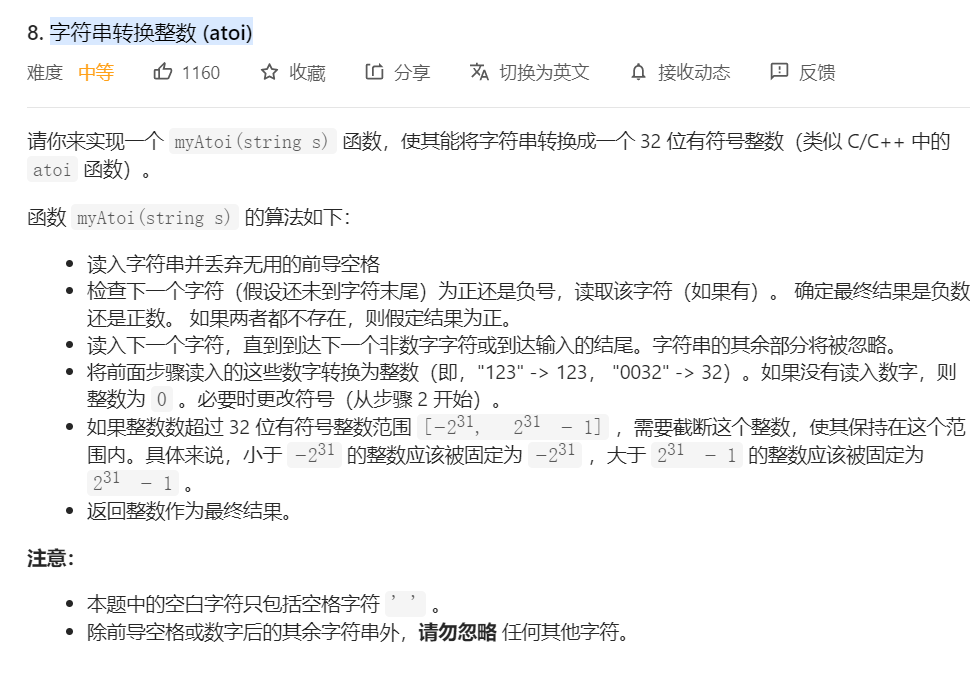``````import re
class Solution:
def myAtoi(self, str: str) -> int:
INT_MAX = 2147483647
INT_MIN = -2147483648
str = str.lstrip()      #清除左边多余的空格
num_re = re.compile(r'^[\+\-]?\d+')   #设置正则规则
num = num_re.findall(str)   #查找匹配的内容
num = int(*num) #由于返回的是个列表，解包并且转换成整数
return max(min(num,INT_MAX),INT_MIN)    #返回值``````

# 200道大数据面试常考Leetcode算法题09-回文数

Leetcode原题：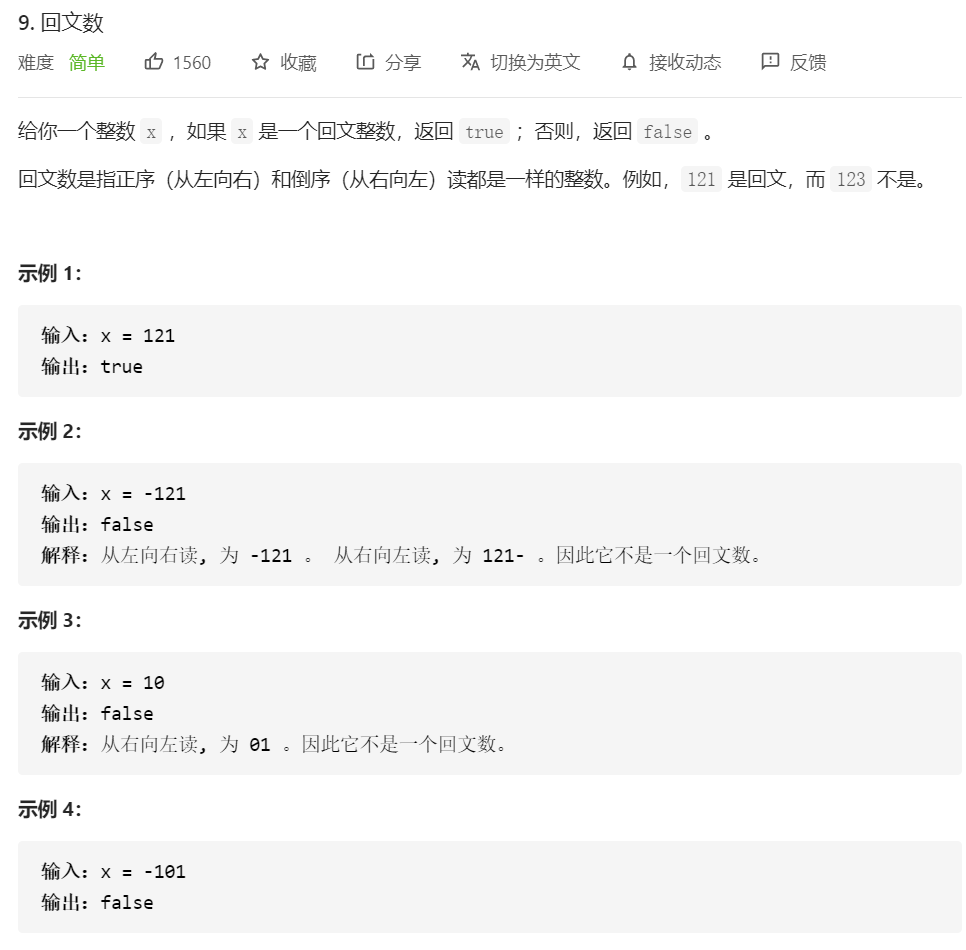``````class Solution:
def isPalindrome(self, x: int) -> bool:
# 判断对正数反转时
if x>=0:
# 整型变成字符串用切片，-1表示反转
ans=int(str(x)[::-1])
# 直接反转对比是否等于原数
if ans == x:
return True
# 其他情况一律不符合
return False``````

# 200道大数据面试常考Leetcode算法题10-正则表达式匹配

Leetcode原题为：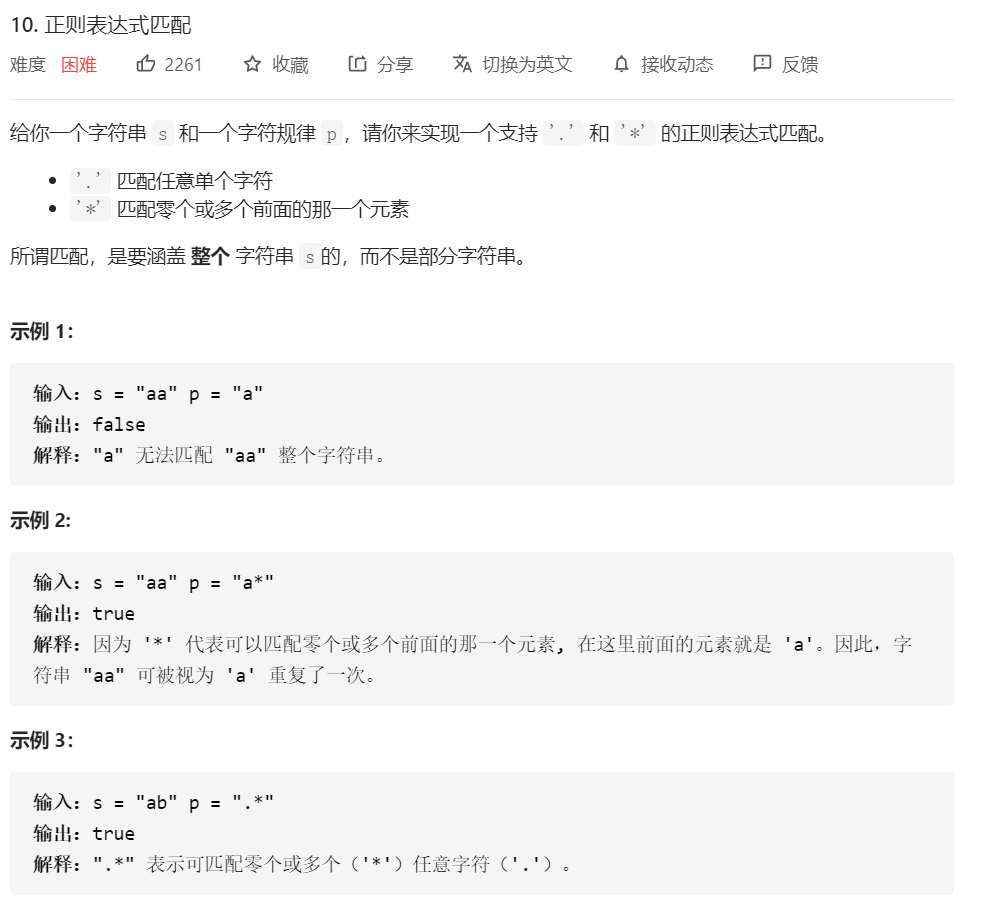题解为：

``````class Solution(object):
def isMatch(self, s, p) -> bool:
if not s and not p:
return True
if len(s) > 0 and not p:
return False
# 当模式中的第二个字符是“*”时：
if len(p) > 1 and p == '*':
# 如果字符串第一个字符跟模式第一个字符匹配，可以有3种匹配方式：
if len(s) > 0 and (s == p or p == '.'):
# 1、模式后移2字符，相当于x*被忽略；
# 2、字符串后移1字符，模式后移2字符；
# 3、字符串后移1字符，模式不变，即继续匹配字符下一位，因为*可以匹配多位；
return self.isMatch(s[:], p[2:]) or self.isMatch(s[1:], p[2:]) or self.isMatch(s[1:], p[:])
# 如果字符串第一个字符跟模式第一个字符不匹配
else:
# 模式后移2个字符，继续匹配。
return self.isMatch(s, p[2:])
# 当模式中的第二个字符不是“*”跟第一个字符和模式中的第一个字符相匹配时：
if len(s) > 0 and (p == s or p == '.'):
# 那么字符串和模式都后移一个字符，然后匹配剩余的。
return self.isMatch(s[1:], p[1:])
# 如果字符串第一个字符和模式中的第一个字符相不匹配，直接返回false。
return False``````

我有话说: